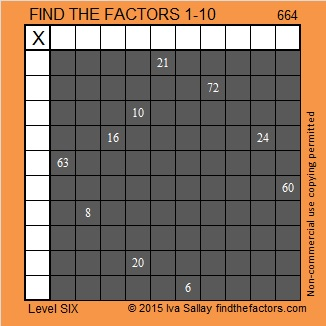# 664 The Back of a Halloween Cat’s Head

664  in base 12 is the palindrome 474. Note that 4(144) + 7(12) + 4(1)= 664.Print the puzzles or type the solution on this excel file: 10 Factors 2015-10-26

—————————————————————————————————

• 664 is a composite number.
• Prime factorization: 664 = 2 x 2 x 2 x 83, which can be written 664 = (2^3) x 83
• The exponents in the prime factorization are 3 and 1. Adding one to each and multiplying we get (3 + 1)(1 + 1) = 4 x 2 = 8. Therefore 664 has exactly 8 factors.
• Factors of 664: 1, 2, 4, 8, 83, 166, 332, 664
• Factor pairs: 664 = 1 x 664, 2 x 332, 4 x 166, or 8 x 83
• Taking the factor pair with the largest square number factor, we get √664 = (√4)(√166) = 2√166 ≈ 25.768197.—————————————————————————————————Ricardo tweeted the solution: